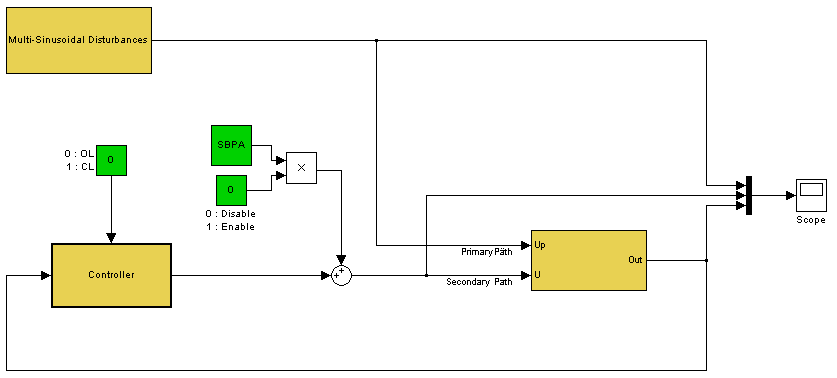Last update: Oct 25, 2013

## 6. Simulator

A discrete time simulator of the active suspension built on Matlab Simulink (Matlab 2007) can be downloaded: bench_sim.zip
A new version of the discrete time simulator of the active suspension built on Matlab Simulink (Matlab 2007) can be downloaded: Simulator_2.zip
The main simulation scheme is given below:The zip file contains the folder bench_sim which includes 3 files: init_simulator_bench.m, bruitbench.mat, and simulator_bench.mdl.
The sampling frequency is 800 Hz.

The new zip file contains the folder Simulator_2 which includes 4 files: Simulator_2.mdl, noise.mat, model_sec.mat, and model_prim.mat.
The sampling frequency is 800 Hz.

The primary path and the secondary path are indicated.
A generator for the PRBS is included in the scheme as well as the generator for the disturbances (up to three sinusoids).
A sample of the system noise in open loop is added to the simulation (a record of 80.000 samples).
The saturation of the actuator is included in the simulator. The corresponding input saturation level is 0.7 V (for both paths).
The control scheme (Controller) should be built around the given simulator using the actual model or another identified one. However, the organizers will test the scheme using the given model before its implementation on the real system.

To start the simulator one should run the init_simulator_bench.m.